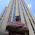Sunday, June 3, 2012

Sudoku Solver - Some Common Questions

Hi,

This is a post to answer some common questions that can arise while dealing with the Sudoku Solver.

Question 1 : What is the need of Smoothing?

Answer : You will understand its need if you see the result without applying Smoothing. Below is the result of Adaptive Threshold without Smoothing.Result of adaptive noise without smoothing
You can see the same result after applying a smoothing:After smoothing
Compare the results. There are lot of noises in the first case. So we have to remove them in the next step which is an extra task.

I just compared number of independent objects found (ie contours ) in both the cases. Below is the result:

First without smoothing:
>>> len(contours)
3109


Next after smoothing:
>>> len(contours)
450


See the difference. Without smoothing, we are dealing with 7 times the number of objects than those found after smoothing. So which one is good?

To know different Smoothing Techniques : Smoothing Techniques in OpenCV

Question 2 : Why adaptive thresholding ? Why not normal thresholding ?

AnswerReason, You will understand when we compare the results of them.

Below is the result, I got using Adaptive Threshold :Result of Adaptive Threshold
Now we apply normal thresholding for a value of 96 ( 96 is the auto threshold value generated by GIMP):Normal thresholding for value = 96
Now see the difference. It is because normal thresholding thresholds the image taken as a whole, while adaptive threshold thresholds the image taking an optimum value for a local neighbourhood.

To know more about thresholding techniques :

Question 3 What is the benefit of filtering contours with respect to area?

Answer : 1) To avoid small noises which has an area less than prescribed value and we are sure it can't be the square

2) It also improves the speed a little bit.

I will show you some performance comparisons below:

A)  We have already calculated number of objects (contours) found, which is 450. Without having any area filter, it process all the 450 contours. For that, you can just change the code as below:

for i in contours:
if area > min_size:
peri = cv2.arcLength(i,True)
approx = cv2.approxPolyDP(i,0.02*peri,True)
if area > max_area and len(approx)==4:
biggest = approx
max_area = area


It checks all the 450 contours for maximum area and it takes an average of 30 ms.

B)  Now we implement a filter for area of 100, as explained in the original code. Then it takes checks only 100 contours and takes only an average of 15 ms. So we get 2X performance.

C)  Now change the value from 100 to 1/4 of the image size. Check the code below:

min_size = thresh.size/4
for i in contours:
if area > min_size:
peri = cv2.arcLength(i,True)
approx = cv2.approxPolyDP(i,0.02*peri,True)
if area > max_area and len(approx)==4:
biggest = approx
max_area = area


Now it checks only one contour,our square, and takes only an average of 3 ms. ie, 10X performance.

Now, although time difference is only 27 ms, it will be highly useful if we implement it in real time.

So, it all depends on how you use it.

1 comment:

1.Hi Abrid!

Thanks a lot for a nice blog. I'm getting a lot for inspiration, to my own CV projects.
When I’m using normal thresholding I’m using ImageJ to determent the values.
I have a quest , how do you determent you adaptive threshold values?

Best,
Lasse